# Creating Formulas to Calculate Values

After you add your data to a worksheet and define ranges to simplify data references, you can create a formula, or an expression that performs calculations on your data. For example, you can calculate the total cost of a customer's shipments, figure the average number of packages for all Wednesdays in the month of January, or find the highest and lowest daily package volumes for a week, month, or year.

To write an Excel 2007 formula, you begin the cell's contents with an equal sign; when Excel 2007 sees it, it knows that the expression following it should be interpreted as a calculation, not text. After the equal sign, type the formula. For example, you can find the sum of the numbers in cells C2 and C3 using the formula =C2+C3. After you have entered a formula into a cell, you can revise it by clicking the cell and then editing the formula in the formula bar. For example, you can change the preceding formula to =C3-C2, which calculates the difference between the contents of cells C2 and C3.

Troubleshooting

If Excel 2007 treats your formula as text, make sure that you haven't accidentally put a space before the equal sign. Remember, the equal sign must be the first character!

Typing the cell references for 15 or 20 cells in a calculation would be tedious, but Excel 2007 makes it easy to handle complex calculations. To create a new calculation, click the Formulas tab on the Ribbon and then, in the Function Library group, click Insert Function. The Insert Function dialog box appears, with a list of functions, or predefined formulas, from which you can choose.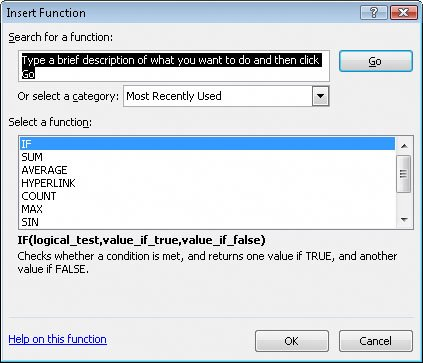The following table describes some of the most useful functions in the list.

Function

Description

SUM

Finds the sum of the numbers in the specified cells

AVERAGE

Finds the average of the numbers in the specified cells

COUNT

Finds the number of entries in the specified cells

MAX

Finds the largest value in the specified cells

MIN

Finds the smallest value in the specified cells

Two other functions you might use are the NOW() and PMT() functions. The NOW() function returns the time the workbook was last opened, so the value will change every time the workbook is opened. The proper form for this function is =NOW(). To update the value to the current date and time, just save your work, close the workbook, and then reopen it.

The PMT() function is a bit more complex. It calculates payments due on a loan, assuming a constant interest rate and constant payments. To perform its calculations, the PMT() function requires an interest rate, the number of months of payments, and the starting balance. The elements to be entered into the function are called arguments and must be entered in a certain order. That order is written PMT(rate, nper, pv, fv, type). The following table summarizes the arguments in the PMT() function.

Argument

Description

rate

The interest rate, to be divided by 12 for a loan with monthly payments

nper

The total number of payments for the loan

pv

The amount loaned (pv is short for present value, or principal)

fv

The amount to be left over at the end of the payment cycle (usually left blank, which indicates 0)

type

0 or 1, indicating whether payments are made at the beginning or at the end of the month (usually left blank, which indicates 0, or the end of the month)

If Consolidated Messenger wanted to borrow \$2,000,000 at a 6 percent interest rate and pay the loan back over 24 months, you could use the PMT() function to figure out the monthly payments. In this case, the function would be written =PMT(6%/12, 24, 2000000), which calculates a monthly payment of \$88,641.22.

You can also use the names of any ranges you defined to supply values for a formula. For example, if the named range NortheastLastDay refers to cells C4:I4, you can calculate the average of cells C4:I4 with the formula =AVERAGE(NortheastLastDay). In previous versions of Excel, you had to type the name into your formula by hand. Excel 2007 enables you to add functions, named ranges, and table references to your formulas more efficiently by using the new Formula AutoComplete capability. Just as AutoComplete offers to fill in a cell's text value when Excel 2007 recognizes that the value you're typing matches a previous entry, Formula AutoComplete offers to fill in a function, named range, or table reference while you create a formula.

As an example, consider a worksheet that contains a two-column table named Exceptions. The first column is labeled Route; the second is labeled Count.You refer to a table by typing the table name, followed by the column or row name in square brackets. For example, the table reference Exceptions[Count] would refer to the Exceptions table's Count column.

To create a formula that finds the total number of exceptions by using the SUM function, you begin by typing =SU. When you type the letter S, Formula AutoComplete lists functions that begin with the letter S; when you type the letter U, Excel 2007 narrows the list down to the functions that start with the letters SU.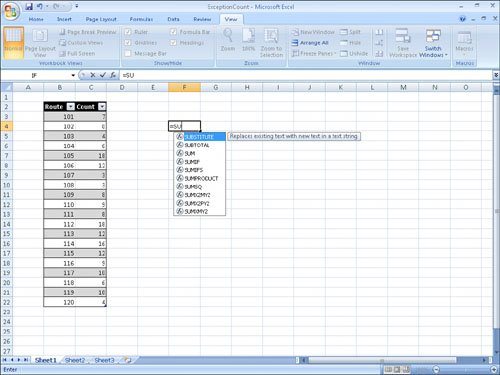To add the SUM function (followed by an opening parenthesis) to the formula, click SUM and then press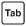. To begin adding the table column reference, type the letter E. Excel 2007 displays a list of available functions, tables, and named ranges that start with the letter E. Click Exceptions and pressto add the table reference to the formula. Then, because you want to summarize the values in the table's Count column, type [Count] to create the formula =SUM(Exceptions[Count]).

If you want to include a series of contiguous cells in a formula, but you haven't defined the cells as a named range, you can click the first cell in the range and drag to the last cell. If the cells aren't contiguous, hold down the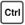key and click the cells to be included. In both cases, when you release the mouse button, the references of the cells you selected appear in the formula.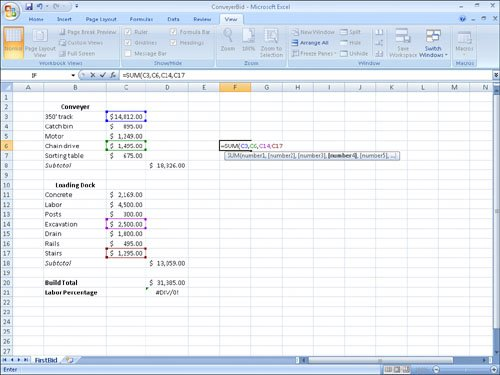After you create a formula, you can copy it and paste it into another cell. When you do, Excel 2007 tries to change the formula so that it works in the new cells. For instance, suppose that you have a worksheet in which cell D8 contains the formula =SUM(C2:C6). Clicking cell D8, copying the cell's contents, and then pasting the result into cell D16 writes =SUM(C10:C14) into cell D16. Excel 2007 has reinterpreted the formula so that it fits the surrounding cells! Excel 2007 knows it can reinterpret the cells used in the formula because the formula uses a relative reference, or a reference that can change if the formula is copied to another cell. Relative references are written with just the cell row and column (for example, C14). If you want a cell reference to remain constant when the formula using it is copied to another cell, you can use an absolute reference. To write a cell reference as an absolute reference, type \$ before the row name and the column number. If you want the formula in cell D16 to show the sum of values in cells C10 through C14 regardless of the cell into which it is pasted, you can write the formula as =SUM(\$C\$10:\$C\$14).

Tip

If you copy a formula from the formula bar, use absolute references or use only named ranges in your formula. Excel 2007 doesn't change the cell references when you copy your formula to another cell.

One quick way to change a cell reference from relative to absolute is to select the cell reference on the formula bar and then press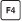. Pressingcycles a cell reference through the four possible types of references:

• Relative columns and rows (for example, C4)

• Absolute columns and rows (for example, \$C\$4)

• Relative columns and absolute rows (for example, C\$4)

• Absolute columns and relative rows (for example, \$C4)

In this exercise, you will create a formula manually, revise it to include additional cells, create a formula that contains a table reference, create a formula with relative references, and change the formula so it contains absolute references.

USE the ITExpenses workbook in the practice file folder for this topic. This practice file is located in the My Documents\Microsoft Press\Excel SBS\Formulas folder.

OPEN the ITExpenses workbook.

 1 If necessary, display the Summary worksheet. Then, in cell F9, type =C4 and press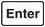. The value \$385,671.00 appears in cell F9. 2 Select cell F9 and then, on the formula bar, erase the existing formula and type =SU. Formula AutoComplete displays a list of possible functions to use in the formula. 3 In the Formula AutoComplete list, click SUM and then press D. Excel 2007 changes the contents of the formula bar to =SUM(. 4 Select the cell range C3:C8, type a right parenthesis (the ) character) to make the formula bar's contents =SUM(C3:C8) and then press. The value \$2,562,966.00 appears in cell F9. 5 In cell F10, type =SUM(C4:C5) and press. 6 Select cell F10 and then, on the formula bar, select the cell reference C4 and press. Excel 2007 changes the cell reference to \$C\$4. 7 On the formula bar, select the cell reference C5, press, and then press. Excel 2007 changes the cell reference to \$C\$5. 8 On the tab bar, click the JuneLabor sheet tab. The JuneLabor worksheet appears. 9 In cell F13, type =SUM(J. Excel 2007 displays JuneSummary, the name of the table in the JuneLabor worksheet. 10 Press. Excel 2007 extends the formula to read =SUM(JuneSummary. 11 Type [, and then, in the Formula AutoComplete list, click [Labor Expense] and press. Excel 2007 extends the formula to read =SUM(JuneLabor[Labor Expense.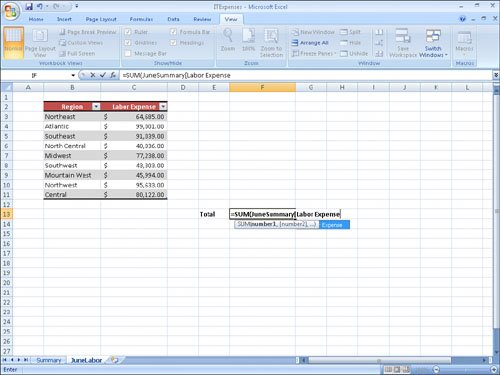12 Type ]) to complete the formula and then press. The value \$637,051.00 appears in cell F13.

CLOSE the ITExpenses workbook.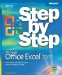MicrosoftВ® Office ExcelВ® 2007 Step by Step (Step By Step (Microsoft))
ISBN: 073562304X
EAN: 2147483647
Year: 2004
Pages: 143
Authors: Curtis Frye

Similar book on Amazon

flylib.com © 2008-2017.
If you may any questions please contact us: flylib@qtcs.net